# The Brachystochrone

(notes by Roberto Bigoni)

## 1. Velocity and duration.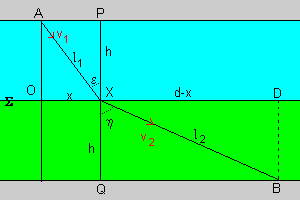A particle moves crossing two contiguous strips of equal thickness h, coloured in blue and green in the figure. The particle enters the blue strip in A, crosses the surface Σ of separation between the two strips in X and exits from the green strip in B. In the blue strip it has constant velocity of intensity v1; in the green strip it has constant velocity of intensity v2. It is assumed that the two velocities lay on the same plane.

To determine the trajectory AXB of minimal duration one can proceed in the following way.

• In the plane of the velocities we place the origin O of an orthogonal cartesian reference frame in the projection of A on Σ; let x be the abscissa of X. Given the projection D of B on Σ, let d be the abscissa of D.
• The segment AX has length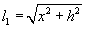and is covered in the time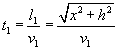• The segment XB has length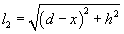and is covered in the time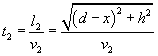• The total duration t of the path AXB is

(1.1)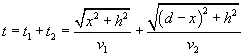• The derivative of t with respect to x is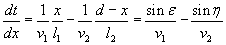and it is null when

(1.2)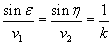where ε and η are the angles formed by the two trajectory segments with the perpendicular to surface Σ in S and k is the constant value of the ratio between the velocities and the sines.

Therefore the trajectory has the shortest duration if in every strip the sine of the angle a segment of trajectory makes with the perpendicular to the surface of separation from another strip is directly proportional to the intensity of the velocity.

## 2. The Snell's law and the Fermat's principle.

From (1.2) one can deduce the proportionality between the sines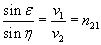.

This is the behaviour of a beam of light when it goes across two optical media in which it has different speeds. The phenomenon of the change of the direction of a light beam is named refraction. The constant n21 is named relative refraction index of the second medium with respect to the first one.

The refraction law, maybe found for the first time by the arab scientist Ibn Sahl around the 10th century, was found in Europe in the 17th century by W. Snell and, maybe independently, by R. Descartes, is now said Snell's law (Descartes' law in France).

It must be observed that, mathematically, the zero value of the first derivative is necessary but not sufficient condition: when the first derivative goes to zero the function may have a maximum, a minimum or a saddle. In general the function has a stationary point.

So we can say that, if a beam of light goes from a point A to a point B, it follows the path which has a stationary duration.

This law was stated in the same 17th century by P. De Fermat in the following way.

If a beam of light crosses consecutively n different media, covering in each of them the length li with velocity vi, the duration of the total path is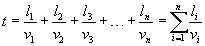The ratio between the speed c of the light in vacuum and the speed vi of the light in an optical medium i is called absolute refraction index ni of the medium. So we can write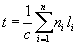Obviously, if a path minimizes t, it minimizes also the sum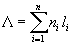called optical path length. If the refraction index varies continuously along the path, the sum must be substituted by an integral: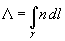The γ below the integral sign represents the path covered by the beam. The Fermat's principle can therefore enounced in the following way: A light beam propagates from the point A to another point B following a stationary optical path length.

This statement is more general than the Snell's law because it can be applied not only to refraction but also to reflection.

## 3. The faster fall.

A particle in a constant gravitational field g falls, if there isn't friction, according the intensity of its velocity v to its height y in a way that can be deduced from the energy conservation principle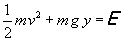In particular, if the particle is initially at rest in the origin O of a cartesian frame of reference Oxy,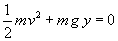, therefore

(3.1)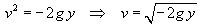Like the optical rays, it must have a trajectory of shortest duration, called brachystochrone (from the ancient greek brachystos='shortest'+ chronos='time'; less correctly many people write brachistochrone).

The determination of the brachystochrone trajectory, tried by Galileo, who wrongly supposed that it is a circumference arc, strongly involved the European scientists between the 1600's and the 1700's; they developed calculation methods even more sophisticated and general. But it can be obtained in a quite simple way.

From (1.2) e (3.1) we have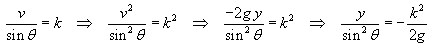(3.2)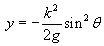The height y has its minimum -h in the point V where sin θ = 1.

In order to obtain the trajectory with the shortest duration we can do the following steps.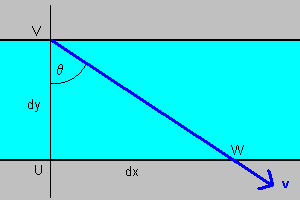• We consider a generic horizontal strip of thickness dy; let v be the intensity of the velocity of the particle in this strip, θ the angle formed by the velocity with the vertical, -h the lowest possible height.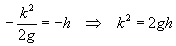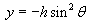• The derivative of y with respect to θ is

(3.3.1)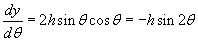• From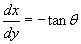we have also

(3.3.2)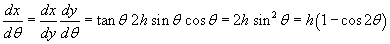• By putting together the (3.3.1) and the(3.3.2),

(3.4)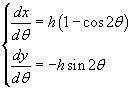The integration of these equations gives the parametric expressions of the coordinates in terms of θ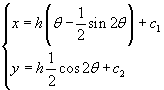In O, θ, x and y are null: this condition allows the determination of c1 and c2.

(3.5)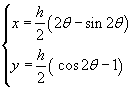## 4. The cycloid.

The (3.5) describe a curve passing though the origin O, through the point S(hπ ; 0), symmetrical with respect to the vertical straight line passing through the point V (the vertex), where it has an absolute minimum.

Such curve is said cycloid. If we graph the (3.5) for θ from 0 to π, making, for example, h=2, with Mathematica (©Wolfram), we obtain

ParametricPlot[{2 t-Sin[2t],Cos[2t]-1},{t,0,Pi},AspectRatio→1,PlotRange→{-2Pi,0}, PlotStyle→{RGBColor[1,0,0]}]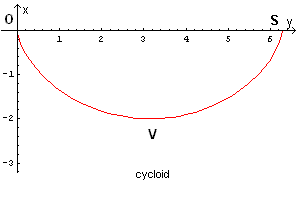The cycloid is the trajectory of a fixed point of a circumference rolling along a straight line.

Your browser does not support the HTML5 canvas tag.

## 5. The duration of the fall to the vertex.

From (3.4) one obtains

(5.1)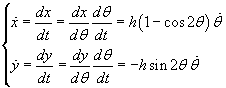The (5.1) are the cartesian components of the velocity: the sum of their squares is the square of the velocity v: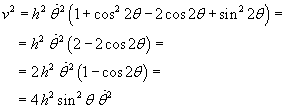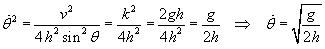The derivative of θ with respect to the time is constant, therefore θ is a linear function of the time and, given θ initially null,

(5.2)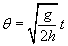By substituting this expression of θ in (3.5) we obtain the trajectory coordinates as functions of time:

(5.3)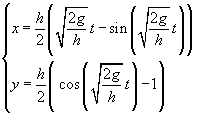From (5.2) one can deduce the time needed to go from O to V: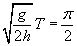(5.4)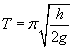A particle which, initially at rest in O, moved by its weight, begins to slide without friction toward V directly on an inclined plane, spends a time Ti greater than T to reach the point V.

From the first of the (3.5) the abscissa of the point V is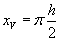The length of the inclined plane is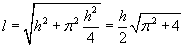If we call δ the angle formed by the plane with an horizontal line, the component γ of the gravity acceleration parallel to the plane is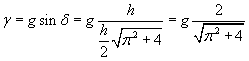From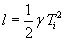we have## 6. The fastest fall to whatever point P.

The equations (3.5) may be practically interesting only if, given whatever point P(α ; -β) (α>0 and β >0), one can calculate the value of the parameter h suitable to reach the point P.

In order to do this, we can act as follows.

• From the second one of the (3.5) we calculate θ as a function of y: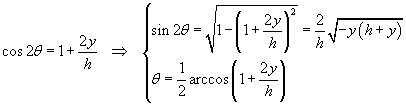• From the first one of the (3.5) we obtain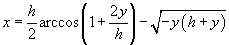• By substituting the coordinates of P to x and y, we obtain the equation

(6.1)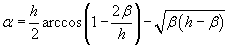Using a numeric method, it is possible to approximate the solution of the (6.1) with respect to h .

• When we have the value of h, from the first one of the (3.5) we can obtain an equation that allows to calculate the value of the parameter θ corresponding to the abscissa of the point P.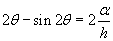Finally, from the (5.2) we obtain the duration of the descent to point P: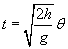The following application shows the brachystochrone trajectory from the point O, where a particle is at rest, to a whatever final point P. It also calculates the duration of the brachystochrone descent and the duration of the descent along an inclined plane from O to P.

input
 abscissa of P (m) ordinate pf P (m)
graph
 duration br. (s) vertex: x vertex: y duration i. p.

last revision: 22/10/2015